Checkout JEE MAINS 2022 Question Paper Analysis : Checkout JEE MAINS 2022 Question Paper Analysis :

# Transversal

In Geometry, we might have come across different types of lines such as parallel lines, perpendicular lines, intersecting lines, and so on. Apart from that, we have another line called transversal. This can be observed when a road crosses two or more roads or a railway line crosses several other lines. These give a basic idea of a transversal. Transversals play an important role in establishing whether two or more other lines in the Euclidean plane are parallel. In this article, you will learn the definition of transversal line, angles made by the transversal with parallel and non-parallel lines with an example.

## Transversal Meaning

A transversal is defined as a line that passes through two lines in the same plane at two distinct points in the geometry. A transversal intersection with two lines produces various types of angles in pairs, such as consecutive interior angles, corresponding angles and alternate angles. A transversal produces 8 angles and this can observed from the figure given below: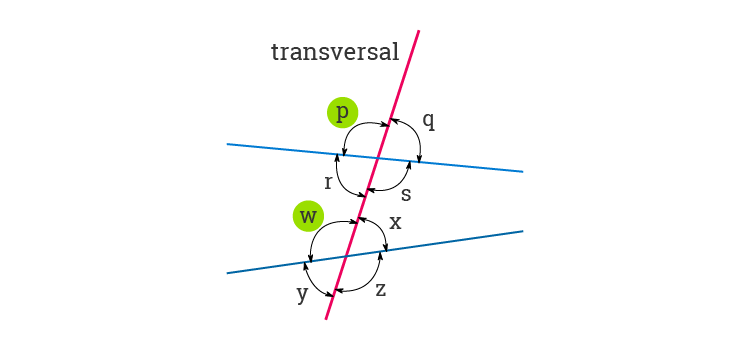Transversal Line Definition

A line that intersects two or more lines at distinct points is called a transversal line.

## Transversal Lines

A transversal line can be obtained by intersecting two or more lines in a plane that may be parallel or non-parallel.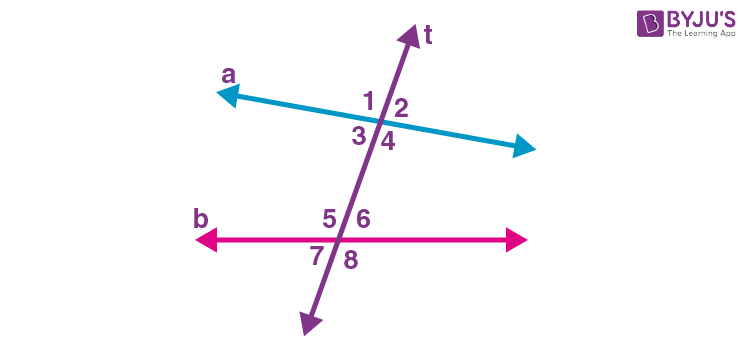In the above figure, line “t” is the transversal of two non-parallel lines a and b.

Also, we can draw two transverse lines for two parallel lines and non-perpendicular lines.

## Transversal and Parallel Lines

In the below given figure, the line RS represents the Transversal of Parallel Lines EF and GH.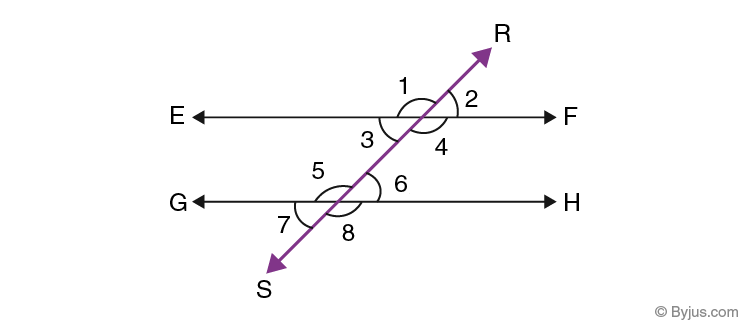### Transversal Angles

The angles made by a transversal can be categorized into several types such as interior angles, exterior angles, pair of corresponding angles, pair of alternate interior angles, pair of alternate exterior angles, and the pairs of interior angles on the same side of the transversal. All these angles can be identified in both the cases, that means parallel and non-parallel lines.

## Transversal Lines and Angles

Let’s understand the angles made by transversal lines with other lines from the below table.Interior angles ∠3, ∠4, ∠5, ∠6 Interior angles ∠3, ∠4, ∠5, ∠6 Exterior angles ∠1, ∠2, ∠7, ∠8 Exterior angles ∠1, ∠2, ∠7, ∠8 Pairs of corresponding angles ∠1 and ∠5, ∠2 and ∠6, ∠3 and ∠7, ∠4 and ∠8 Pairs of corresponding angles ∠1 and ∠5, ∠2 and ∠6, ∠3 and ∠7, ∠4 and ∠8 Pairs of alternate interior angles ∠3 and ∠6, ∠4 and ∠5 Pairs of alternate interior angles ∠3 and ∠6, ∠4 and ∠5 Pairs of alternate exterior angles ∠1 and ∠8, ∠2 and ∠7 Pairs of alternate exterior angles ∠1 and ∠8, ∠2 and ∠7 Pairs of interior angles on the same side of the transversal ∠3 and ∠5, ∠4 and ∠6 Pairs of interior angles on the same side of the transversal ∠3 and ∠5, ∠4 and ∠6

From the above table, we can say that the angles associated with the transversal will be the same in pairs in parallel and non-parallel lines.

### Transversal Properties

Some of the properties of transversal lines with respect to the parallel lines are listed below.

• If two parallel lines are cut by a transversal, each pair of corresponding angles are equal in measure.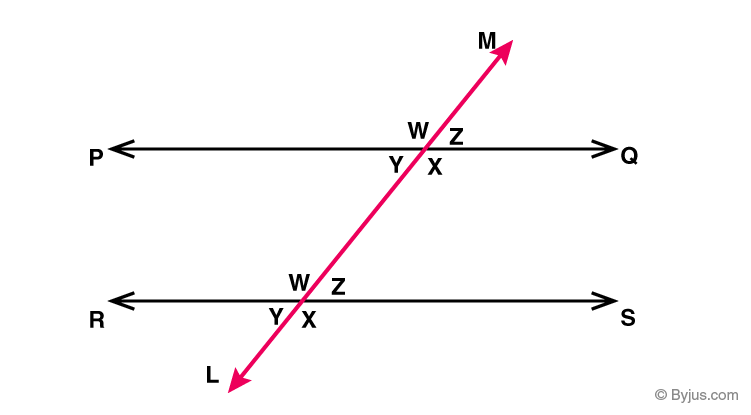Here,

LM is the transversal made by the parallel lines PQ and RS such that:

The pair of corresponding angles that are represented with the same letters are equal.

• If two parallel lines are cut by a transversal, each pair of alternate interior angles are equal.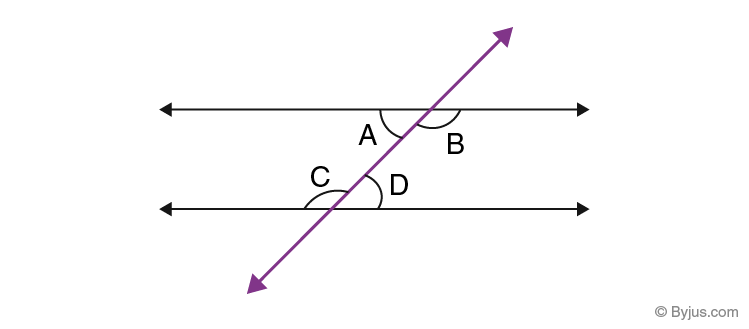Here, ∠A = ∠D and ∠B = ∠C

• If two parallel lines are cut by a transversal, then each pair of interior angles on the same side of the transversal are supplementary, i.e. they add up to 180 degrees.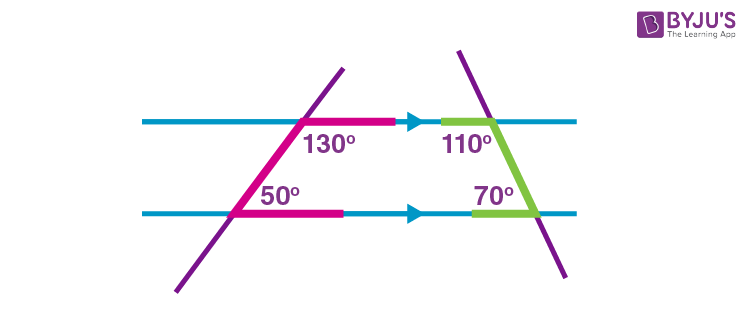This property can also be written for a single transversal line.Here,

∠3 + ∠5 = 180° and ∠4 + ∠6 = 180°

### Transversal Example

Let’s have a look at the solved example given below:

Question: In the given figure, AB and CD are parallel lines intersected by a transversal PQ at L and M, respectively. If ∠CMQ = 60°, find all other angles.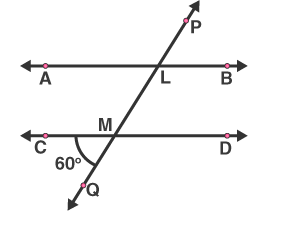Solution:

A pair of angles in which one arm of both the angles is on the same side of the transversal and their other arms are directed in the same sense is called a pair of corresponding angles.

Thus, the corresponding angles are equal.

∠ALM = ∠CMQ = 60° {given}

We know that vertically opposite angles are equal.

∠LMD = ∠CMQ = 60° {given}

And

∠ALM = ∠PLB = 60°

Here, ∠CMQ + ∠QMD = 180° are the linear pair

On rearranging we get,

∠QMD = 180° – 60° = 120°

Also, the corresponding angles are equal.

∠QMD = ∠MLB = 120°

Now,

∠QMD = ∠CML = 120° {vertically opposite angles}

∠MLB = ∠ALP = 120° {vertically opposite angles}

## Frequently Asked Questions on Transversal

### What is transversal property?

When a transversal is formed by intersecting two parallel lines, then the following properties can be defined.

1. If a transversal cuts two parallel lines, each pair of corresponding angles are equal in measure.

2. If a transversal cuts two parallel lines, each pair of alternate interior angles are equal.

3. If a transversal cuts two parallel lines, then each pair of interior angles on the same side of the transversal are supplementary.

### What does transversal mean in geometry?

In geometry, a transversal is a line that intersects two or more lines at distinct points.

### What is the transversal theorem?

Various theorems are defined for transversal, such as:

1. If a transversal intersects two parallel lines, then each pair of corresponding angles is equal.

2. If a transversal intersects two lines such that a pair of corresponding angles are equal, then the two lines are parallel to each other.

3. If a transversal intersects two lines such that a pair of alternate interior angles are equal, then the two lines are parallel.

4. If a transversal intersects two parallel lines, then each pair of interior angles on the same side of the transversal is supplementary.

### How do you identify a transversal?

We can quickly identify the transversal since it crosses two or more lines at different points.

### What is the symbol for transversal?

We do not have any particular symbol to represent a transversal in maths.

### How do you label a transversal?

We can label a transversal similar to other lines in geometry, which means using the English alphabets. For example, line PQ is the transversal of two lines AB and CD.

### What is the symbol for similar to?

The symbol that denotes the similarity is ~. This can be read as “is similar to”.